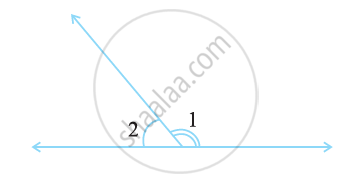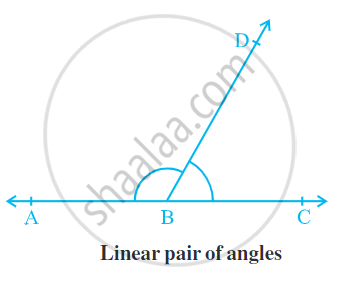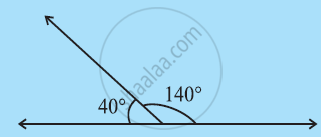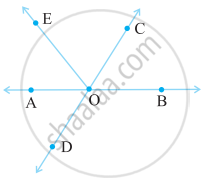# Related Angles - Concept of Linear Pair

#### definition

Linear pair: A linear pair is a pair of adjacent angles whose non-common sides are opposite rays.

# Linear Pair:

• A linear pair is a pair of adjacent angles whose non-common sides are opposite rays.• The opposite rays (which are the non-common sides of ∠ 1 and ∠ 2) form a line. Thus, ∠1 + ∠2 amounts to 180°.

• The angles in a linear pair are supplementary.

• A pair of supplementary angles form a linear pair when placed adjacent to each other.

• Example, 40° + 140° = 180°• If the non-common arms BA and BC form a line then ∠ ABD and ∠ DBC are called linear pairs of angles.

Some more Examples,#### Example

In the given figure, Identify three linear pairs.Linear pairs are (∠AOE, ∠EOB), (∠AOC, ∠COB), (∠COB, ∠BOD).

If you would like to contribute notes or other learning material, please submit them using the button below.

### Shaalaa.com

What is Linear Pair? [00:07:43]
S
0%